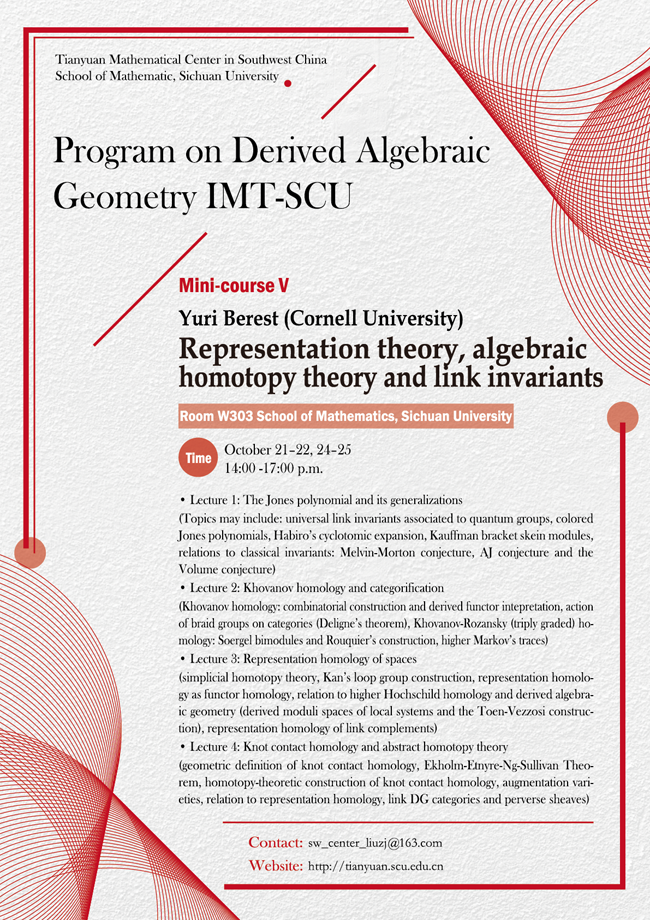2019 Thematic Program (IV)

### Representation theory, algebraic homotopy theory and link invariants

#### Venue: W303  School of Mathematics, Sichuan University#### SPEAKER

Yuri Berest (Cornell University)

#### ABSTRACT

Deep connections between representation theory and low-dimensional topology became apparent in the late 80s with the discovery of Jones' polynomial and its generalizations related to quantum groups. Since then, much work has been done to clarify the relation of these new ‘quantum’ invariants to classical geometric invariants of 3-manifolds, such as representation varieties of fundamental groups.

In recent years, new types of connections and, in fact, an entirely new paradigm of the interactions between representation theory and topology have emerged. On the algebraic side, the focus has shifted to developing a higher (categorical) version of representation theory, where classical linear representations are replaced by additive (abelian or triangulated) categories and the principal objects of study are ‘categorified’ quantum groups and related structures (such as affine and double affine Hecke algebras). On the topological side, the main interest is in understanding various new homology theories associated to knots and links (such as Khovanov homology and triply graded link homology) and their relation to classical structures in homotopy theory and geometric topology (such as braids and mapping class groups).

Yet another new, very interesting class of homology theories of links originates from symplectic topology. This includes the Legendrian Floer homology of Ozsváth, Szabó and the so-called knot contact homology (introduced by L. Ng and studied by Etnyre, Ekholm, Sullivan and others in recent years). Despite efforts of many mathematicians, a precise relation between these geometric homology theories and those coming from representation theory and classical topology remains quite a mystery (although there are some very intriguing conjectures in this direction).

The goal of these lectures is to give a survey of various connections --both old and new -- between representation theory, classical homotopy theory and low-dimensional topology aimed at graduate students. Although the required background and the level of presentation will vary from lecture to lecture (some topics will be covered in a systematic way while others will be touched upon only briefly), we will try to make the overall discussion accessible for the beginning graduate students. A tentative plan is as follows:

• Lecture 1: The Jones polynomial and its generalizations

(Topics may include: universal link invariants associated to quantum groups, colored Jones polynomials, Habiro's cyclotomic expansion, Kauffman bracket skein modules, relations to classical invariants: Melvin-Morton conjecture, AJ conjecture and the Volume conjecture)

• Lecture 2: Khovanov homology and categorification

(Khovanov homology: combinatorial construction and derived functor intepretation, action of braid groups on categories (Deligne's theorem), Khovanov-Rozansky (triply graded) homology: Soergel bimodules and Rouquier's construction, higher Markov's traces)

• Lecture 3: Representation homology of spaces

(simplicial homotopy theory, Kan's loop group construction, representation homology as functor homology, relation to higher Hochschild homology and derived algebraic geometry (derived moduli spaces of local systems and the Toen-Vezzosi construction), representation homology of link complements)

• Lecture 4: Knot contact homology and abstract homotopy theory

(geometric definition of knot contact homology, Ekholm-Etnyre-Ng-Sullivan Theorem, homotopy-theoretic construction of knot contact homology, augmentation varieties, relation to representation homology, link DG categories and perverse sheaves)